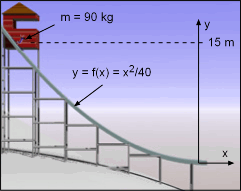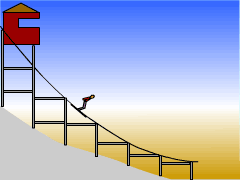Ch 2. Particle Force and Acceleration Multimedia Engineering Dynamics Rect.Coord. Normal/Tang. Coord. PolarCoord. Orbital Mechanics Computational Mechanics
 Chapter - Particle - 1. General Motion 2. Force & Accel. 3. Energy 4. Momentum - Rigid Body - 5. General Motion 6. Force & Accel. 7. Energy 8. Momentum 9. 3-D Motion 10. Vibrations Appendix Basic Math Units Basic Equations Sections Search eBooks Dynamics Fluids Math Mechanics Statics Thermodynamics Author(s): Kurt Gramoll ©Kurt GramollDYNAMICS - CASE STUDY IntroductionCoordinate System Just prior to the Winter Olympics, the Olympic committee must erect a 15-meter ski jump. If the jump is not sturdy enough, the force of the skier could cause the jump to collapse. What is known: The shape of the ski jump is described by the equation y = f(x) = x2/40   (m) The largest contestant has a total mass of 90 kg, including equipment. The skier starts from rest at a height of 15 m. Friction and the size of the skier and skis may be ignored.Problem Graphic Question At the end of the jump, what is the normal force exerted on the skier by the jump? What is the total acceleration of the skier at the end of the jump? Approach Use the principle of conservation of energy (covered in more detail in the following chapter) to find the skier's velocity at the end of the jump. Apply Newton's Second Law, using the equations for normal and tangential coordinates developed in the Curvilinear Motion: Normal/Tangential Coordinates section of previous chapter.

Practice Homework and Test problems now available in the 'Eng Dynamics' mobile app
Includes over 400 problems with complete detailed solutions.
Available now at the Google Play Store and Apple App Store.